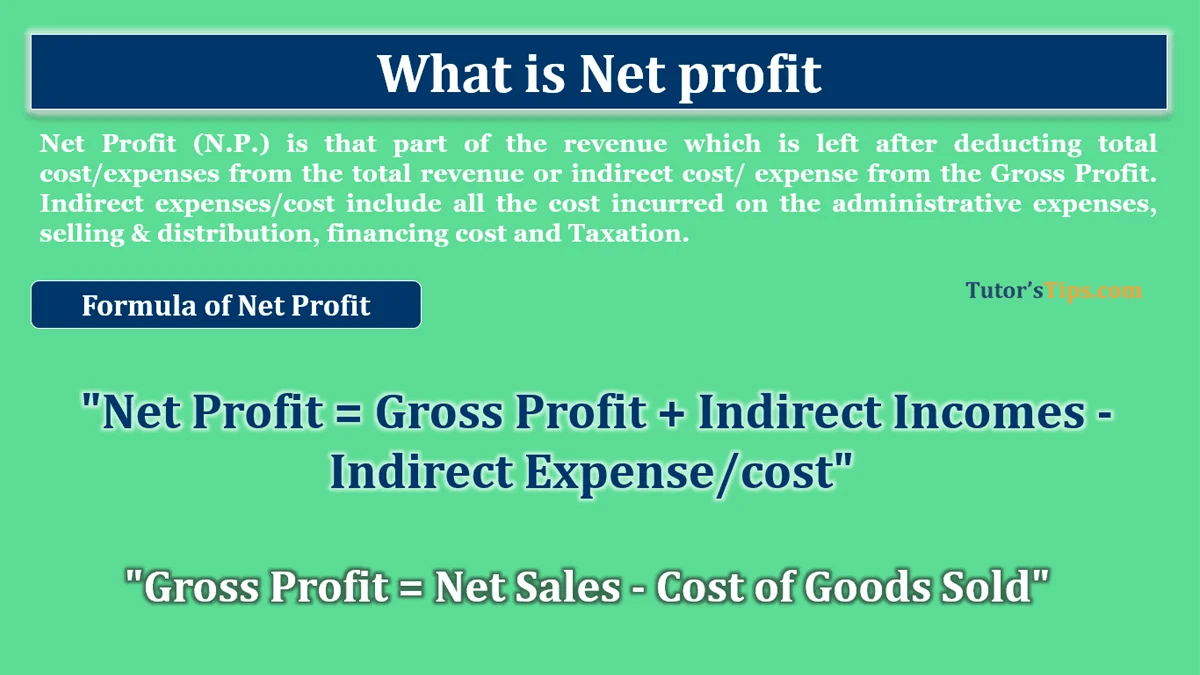# What is Net profit – Explanation with ExamplesWhat-is-Net-Profit

Net Profit (N.P.) is that part of the revenue which is left after deducting total cost/expenses from the total revenue or indirect cost/ expense from the Gross Profit. Indirect expenses/cost include all the cost incurred on the administrative expenses, selling & distribution, financing cost and Taxation. It is also known as Earning After Interest and Taxation(EAIT)

### The Formula of Net Profit : –

“Net Profit = Gross Profit + Indirect Incomes – Indirect Expense/cost”

#### Example 1 : –

Gross Profit of the year is Rs 18,5000 and  Further Mr X paid a salary to his employees 2,500/-, shop rent 1,000 and Shop lighting 200. Now calculate the Net Profit earned by Mr X.

Solution: –

Net Profit = Gross Profit + Indirect Income – Indirect Cost/ Expenses

We already got Gross Profit in the question = 18,500/-

SO,  18500 – 2500 -1000 – 200

N.P. = 14,800/-

#### Example 2 : –

Gross Profit of the year is Rs. 5,00,000, Further Mr Y paid a salary to his employees 92,500/-, Electricity charges Rs. 25,000 and Depreciation of Building Rs 50,000. In addition to that, he has received the Rent from the Tenant for the let-out building Rs. 50,0000. Mr  Now calculates the N.P. earned by Mr Y.

Solution: –

N.P = Gross Profit + Indirect Income – Indirect Cost/ Expenses

We already got Gross Profit in the question = 5,00,000/-

SO,  Total of Indirect Income =  50,000/-

Total of Indirect Expenses/cost =  92,500 + 25,000 + 50,000

= 1,67,500/-

N.P. = 5,00,000 + 50,000 – 1,67,500

N.P. = 3,82,500/-

#### Example 3 : –

Gross Profit of the year is Rs. 2,00,000, Further Mr Z paid a salary to his employees 80,000/-, Freight on Sales of goods Rs. 5,000, Depreciation of all assets Rs 20,000, Interest on loan Rs 10,000 and Taxes Rs 15,000. In addition to that, he has received the Rent from the Tenant for the let-out building Rs. 25,000. Mr  Now calculates the N.P. (EAIT) earned by Mr Z.

Solution: –

N.P = Gross Profit + Indirect Income – Indirect Cost/ Expenses

We already got Gross Profit in the question = 2,00,000/-

SO,  Total of Indirect Income =  25,000/-

Total of Indirect Expenses/cost =  80,000 + 5,000 + 20,000 + 10,000 + 15,000

= 1,30,000/-

N.P. = 2,00,000 + 25,000 – 1,30,000

N.P. = 95,000/-

Check out the Difference between Gross and Net Profit.

Difference between Gross profit and Net Profit

You can also read the following topics: –